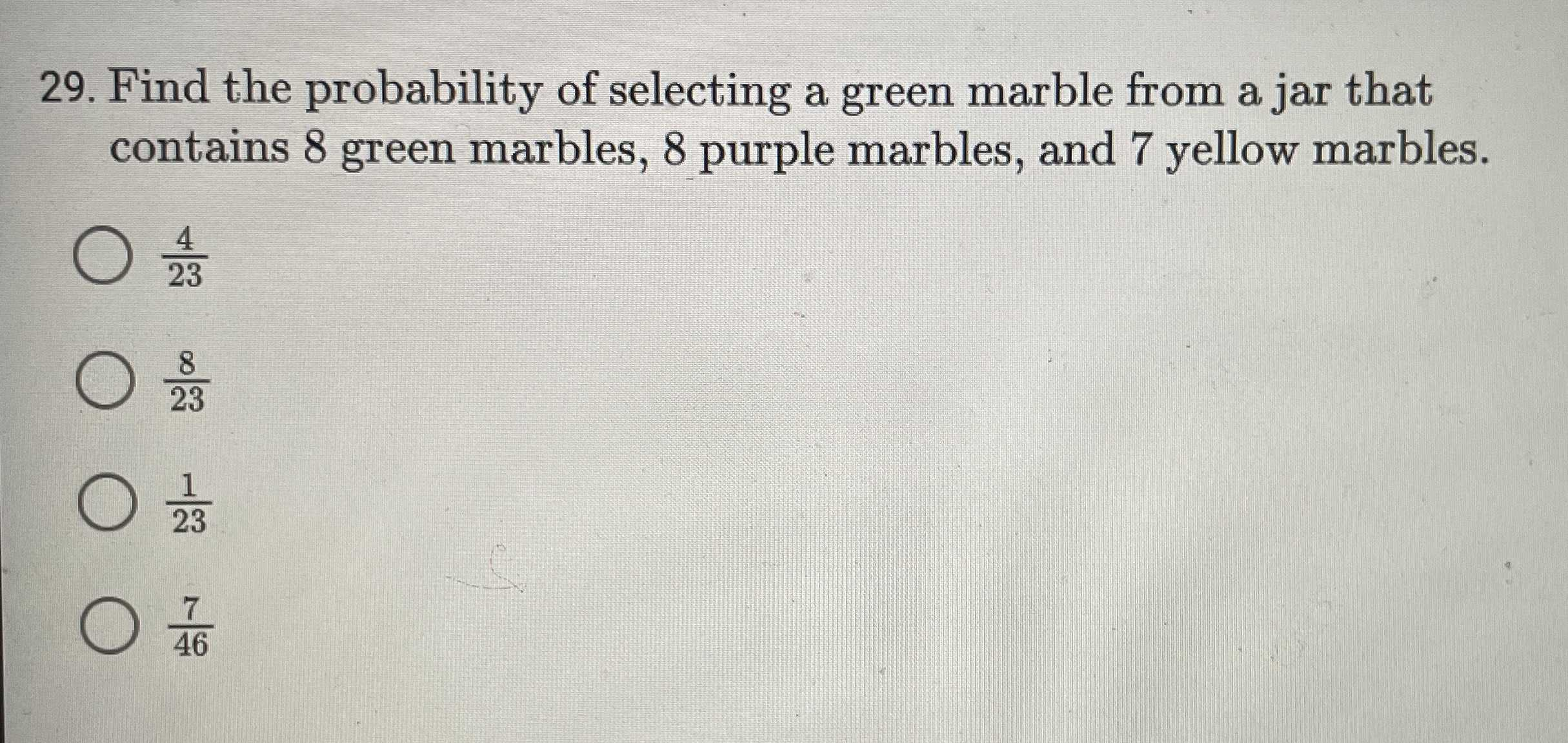### Still have math questions?

Arithmetic
Question29. Find the probability of selecting a green marble from a jar that contains $$8$$ green marbles, $$8$$ purple marbles, and $$7$$ yellow marbles.

$$\frac { 4 } { 23 }$$

$$\frac { 8 } { 23 }$$

$$\frac { 1 } { 23 }$$

$$\frac { 7 } { 46 }$$

the probability of selecting a green marble  from the jar=$$\frac{8}{23}$$